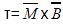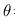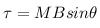Courses

# Test: Magnetism And Matter

## 30 Questions MCQ Test Physics Class 12 | Test: Magnetism And Matter

Description
This mock test of Test: Magnetism And Matter for JEE helps you for every JEE entrance exam. This contains 30 Multiple Choice Questions for JEE Test: Magnetism And Matter (mcq) to study with solutions a complete question bank. The solved questions answers in this Test: Magnetism And Matter quiz give you a good mix of easy questions and tough questions. JEE students definitely take this Test: Magnetism And Matter exercise for a better result in the exam. You can find other Test: Magnetism And Matter extra questions, long questions & short questions for JEE on EduRev as well by searching above.
QUESTION: 1

### Which of the following statements about earth's magnetism is correct

Solution:

According to recent researches the magnetic field of earth is considered due to a large bar magnet situated in earth's core.
It is considered that the north pole of this large magnet is situated at the geographical south of earth and vice versa and as the magnetic field due to a bar magnet is from north pole to south pole of the maget thus the earth's magnetic field is considered from geographical south to geographical north which are respectively north and south poles of the bar magnet.

QUESTION: 2

### magnetic permeability of the substance μ is

Solution:

μ = μ0μr. μ is the permeability of medium, μ0 is permeability of free space, and μr is relative permeability of the medium.

QUESTION: 3

### A toroid wound with 60.0 turns/m of wire carries a current of 5.00 A. The torus is iron, which has a magnetic permeability of μm=5000μ0 under the given conditions. H and B inside the iron are

Solution:

B=B0+Bm
= μ0nI+ μmnI
=nI(μ+ μm)
=60x5(5000μ0+ μ0)
B=300(5001μ0)
B=1.88T
H=B/μm
=300x5001μ0/5000μ
=300AT

QUESTION: 4

A bar magnet of magnetic moment 1.5 J/T lies aligned with the direction of a uniform magnetic field of 0.22 T. What is the amount of work required by an external torque to turn the magnet so as to align its magnetic moment opposite to the field direction?

Solution:

Work required to turn the dipole is given by
W=MB[cosθi- cosθf]
Here θi is the initial angle made by a dipole with a magnetic field and   is the final angle made by a dipole with a magnetic field.
magnetic moment is normal to the field direction.
so, θi=0 and θf=90
W = 1.5 × 0.22 [ cos0° - cos90° ]
W = 0.33J

magnetic moment is opposite to the field direction.
so, θi=0 and θf=180
now, W = 1.5 × 0.22 [ cos0° - cos180°]
= 0.33 [ 1 - (-1) ]
= 0.66 J

QUESTION: 5

In the magnetic meridian of a certain place, the horizontal component of the earth’s magnetic field is 0.26G and the dip angle is 60o. What is the magnetic field of the earth at this location

Solution:

The earth's magnetic field is Be​ and its horizontal and vertical components are He​ and Hv​
cosθ= He​​/Be
∴cos60o= (​0.26×10−4​/ Be )T
⇒Be​=(​0.26×10−4)/ (½)​=0.52×10−4T=0.52G

QUESTION: 6

Which of the following statements about bar magnets is correct ?

Solution:

Explanation:As magnetic monopole does not exist. If we split the bar magnet into two pieces  each part will have its own north and south pole.

QUESTION: 7

Magnetic material differences are explained by

Solution:

All substances show some kind of magnetic behaviour. After all, they are made up of charged particles: electrons and protons. It is the way in which electron clouds arrange themselves in atoms and how groups of these atoms behave that determines the magnetic properties of the material. The atom (or group of atoms) in effect becomes a magnetic dipole or a mini bar magnet that can align according to the magnetic field applied. The net effect of all these dipoles determines the magnetic properties of the Magnetic Materials.

QUESTION: 8

A cube-shaped permanent magnet is made of a ferromagnetic material with a magnetization 500M of about The side length is 20 cm. Magnetic dipole moment of the magnet is.

Solution:

Correct Answer :- C

Explanation : Magnetic Moment = Magnetization × Volume

Side of cube = 20 cm

= 0.2 m

= 500 × 0.2 × 0.2 × 0.2

= 4Am2

QUESTION: 9

A bar magnet of magnetic moment 1.5 J/T lies perpendicular to the direction of a uniform magnetic field of 0.22 T. What is the torque acting on it?

Solution:=1.5x0.22
=15x22x10-3
=330x10-3
Τ=0.33J

QUESTION: 10

A short bar magnet placed in a horizontal plane has its axis aligned along the magnetic north-south direction. Null points are found on the axis of the magnet at 14 cm from the centre of the magnet. The earth’s magnetic field at the place is 0.36 G and the angle of dip is zero. What is the total magnetic field on the normal bisector of the magnet at the same distance as the null–point (i.e., 14 cm) from the centre of the magnet? (At null points, field due to a magnet is equal and opposite to the horizontal component of earth’s magnetic field.)

Solution:

At null point (and along the axis), earth's magnetic field and bar's magnetic field are opposite in direction.
On the equatorial line, the bar's magnetic field is opposite in direction to its field on the axis. Hence, on the equatorial line, the two fields add up.
As the null points are on the axis of the bar magnet, therefore,
B1= (μ0/4π)(2M/d3)=H
On the equatorial line of magnet at same distance(d), field due to the magnet is
B2= (μ0/4π)(M/d3)=B1/2=H/2
Therefore, total magnetic field at this point on equatorial line is
B=B2+H=3H/2=3×0.36G/2=0.54G

QUESTION: 11

When iron filings are sprinkled on a sheet of glass placed over a short bar magnet then,

Solution:

Explanation:North pole act as positive end and South pole act as negative end. The iron fillings in the presence of magnetic field  gets magnetized and form a tiny magnet which then gets  attracted to the poles of bar magnet. North pole of bar magnet attracts south pole of tiny magnet and vice-versa. So all the iron fillings are arranged as the magnetic field lines of bar magnet.

QUESTION: 12

Diamagnetic substances are

Solution:

When a diamagnetic material is placed in an external magnetic field the spin motion of electrons is so modified that the electrons which produce the moments in the direction of the external field show down while the electrons which produce magnetic moments in opposite directions get accelerated.
Thus, a net magnetic moment is induced in the opposite direction of the applied magnetic field. Hence the substance is magnetized opposite of the external field. Thus, it moves from stronger. Weaker parts of the magnetic.

QUESTION: 13

A cube-shaped permanent magnet is made of a ferromagnetic material with a magnetization 8 * 105 M of about The side length is 2 cm. Magnetic field due to the magnet at a point 10 cm from the magnet along its axis is

Solution:

Correct Answer :- d

Explanation : μtotal = MV

= (8*105)(2*10-2)

= 6A m2

Magnetic field on the axis of a current loop with magnetic material μtotal is:

B = μμtotal / (2π(x2 + a2)1/2

B = (4π*10-7)(6) / [2π(0.1)]

= 1 * 10-3 T

= 0.001 T

QUESTION: 14

A bar magnet of magnetic moment 1.5 J/T lies parallel to the direction of a uniform magnetic field of 0.22 T. What is the torque acting on it?

Solution:

Answer :-

Solution :- torque when=180°= 1.5 × 0.22 × sin 180°

= 0.33 × 0 = 0 Nm

QUESTION: 15

A short bar magnet of magnetic moment 5.25×10−2JT−1 is placed with its axis perpendicular to the earth’s field direction. At what distance from the centre of the magnet, the resultant field is inclined at 45 with earth’s field on its normal bisector . Magnitude of the earth’s field at the place is given to be 0.42 G. Ignore the length of the magnet in comparison to the distances involved.

Solution:

According to the question, the resultant field is inclined at 45o with earth's magnetic field.
tanθ=BH​​/B
θ=45o
tanθ=1=BH​​/B
BH​=B= μo​M​/4πr3
Given, B= 0.42×10−4T
M=5.25×10−2J/T
Therefore,0.42+10−4=10−7×[(5.25×10−2)/r3]​
r3=12.5×10−5=125×10−6
r=5×10−2m = 5 cm

QUESTION: 16

Which of the following statements about magnetic field lines true?

Solution:

Magnetic poles exist in pairs. It is not possible to isolate a north pole or a south pole. Magnetic field lines start from the north pole and go to the south pole and return to the north pole. They form continuous closed loops unlike electric lines of force which do not as an electric monopole, a single charge does exist.

QUESTION: 17

For diamagnetic materials

Solution:

It is because when we make a group of electrons inside the material it is called domain. So when we apply an external magnetic field on the material. Those domains start to align themselves in the direction of the magnetic field. BUT NOT ALL DOMAINS ALIGN THEMSELVES IN THAT DIRECTION. So option D is correct.

QUESTION: 18

permeability of a paramagnetic material is expected to decrease with increasing temperature because

Solution:

When a parametric material comes close to a magnetic field the atoms will align with the magnetic field which causes another magnetic field. Permeability is the total magnetic field divided by the original magnetic field. Increase in temperature makes it harder for the atoms to align which decreases the strength of the second field. This results in a lower total magnetic field (numerator) and thus lower permeability.

QUESTION: 19

A magnetic needle free to rotate in a vertical plane parallel to the magnetic meridian has its north tip pointing down at 220 with the horizontal. The horizontal component of the earth’s magnetic field at the place is known to be 0.35 G. Determine the magnitude of the earth’s magnetic field at the place.

Solution:

We know the horizontal component of earth magnetic field H=R cos θ
Where θ=angle of dip=220 and the value of H=0.35G
So, putting the values respectively R= H/cosθ=0.35/cos22=0.35/0.927=0.377=0.38G

QUESTION: 20

A Rowland ring of mean radius 15 cm has 3500 turns of wire wound on a ferromagnetic core of relative permeability 800. What is the magnetic field B in the core for a magnetising current of  12 A ?

Solution:

Given data,
Rowland ring is toroid with a core of magnetic material,
Radius of the ring r=15cm
Number of turns of the wire N=3500
Relative permeability of the core,μr​=800
Magnetising current, I=1.2A
Magnetic field, B=?
Using the formula for the magnetic field of a toroid,
B=μ0​nI
Number of turns per unit length, n=N/2πr=3500/​(2π×15×10−2)
Therefore,
B=μ0​μr​nI
The magnetic field is given by the relation,
B= μr​μ0​IN​/2πr
= (800×4π×10−7×1.2×3500)/​ 2π×0.15 =4.48T

QUESTION: 21

In which case of comparing solenoid and bar magnet there is no exact similarity?

Solution:

A bar magnet may be thought of as a large number of circulating currents in analogy with a solenoid. On cutting a bar magnet in half we get two smaller solenoids with weaker magnetic properties.The field lines remain continuous, emerging from one face of the solenoid and entering into the other face. (i.e. similar magnetic fields). The magnetic moment of a bar magnet is also equal to the magnetic moment of an equivalent solenoid.

QUESTION: 22

For paramagnetic materials

Solution:

For paramagnetic materials orbital and spin magnetic moments of the electrons are of the order of bohr magneton.
Paramagnetic materials have some unpaired electrons due to these unpaired electrons the net magnetic moment of all electrons in an atom is not added up to zero. Hence atomic dipole exists in this case. On applying external magnetic field the atomic dipole aligns in the direction of the applied external magnetic field. In this way, paramagnetic materials are feebly magnetized in the direction of the magnetizing field.

QUESTION: 23

If a magnet is suspended over a container of liquid air, it attracts droplets to its poles. The droplets contain only liquid oxygen and no nitrogen because

Solution:

If a magnet is suspended over a container of liquid air, it attracts droplets to its poles. The droplets contain only liquid oxygen; even though nitrogen is the primary constituent of air, it is not attracted to the magnet. Explain what this tells you about the magnetic susceptibilities of oxygen and nitrogen, and explain why a magnet in ordinary, room-temperature air doesn’t attract molecules of oxygen gas to its poles.

QUESTION: 24

A short bar magnet has a magnetic moment of 0.48 J/T .Magnetic field produced by the magnet at a distance of 10 cm from the centre of the magnet on the axis has a direction and magnitude of .

Solution:

Here M=0.48JT−1,d=10cm=0.1m
On axis,
B= (μ0​​/4π)(2M/d3)​=10−7×(2×0.48/0.13)​=0.96×10−4T

QUESTION: 25

A compass needle free to turn in a horizontal plane is placed at the centre of circular coil of 30 turns and radius 12 cm. The coil is in a vertical plane making an angle of 450 with the magnetic meridian. When the current in the coil is 0.35 A, the needle points west to east. Determine the horizontal component of the earth’s magnetic field at the location

Solution:

The needle will point along W-E if the result of the earth’s magnetic field and magnetic field due to the coil have a resultant in the W-E direction. This happens if B cos 45o=Earth's field
⟹ (μ0​nI​/2r) cos45o=(4π×10−7×30×0.35​)/(2×0.12) ×(1/√2)​​=0.39G=Earth's magnetic field.

QUESTION: 26

Far axial magnetic field of a solenoid as well as bar magnet vary

Solution:

For a short Bar Magnet, the magnetic induction at a point on the axis at a distance r from centre is given by the formula
B= (μ0/4π​​) (2M​/r3)
⇒ B= K​/ r3
⇒ B∝1/r3
The correct answer is option D.

QUESTION: 27

In Ferromagnetic materials

Solution:

A ferromagnetic substance contains permanent atomic magnetic dipoles that are spontaneously oriented parallel to one another even in the absence of an external field. The magnetic repulsion between two dipoles aligned side by side with their moments in the same direction makes it difficult to understand the phenomenon of ferromagnetism. It is known that within a ferromagnetic material, there is a spontaneous alignment of atoms in large clusters. A new type of interaction, a quantum mechanical effect known as the exchange interaction, is involved. A highly simplified description of how the exchange interaction aligns electrons in ferromagnetic materials is given here.

QUESTION: 28

which of the following features of atomic structure determine whether an element is diamagnetic or paramagnetic

Solution:

Spin and Orbital angular momentum arise due to the presence of unpaired electrons. If there are unpaired electrons, these momenta will be there otherwise not.

QUESTION: 29

A toroidal solenoid with 500 turns is wound on a ring with a mean radius of 2.90 cm. Find the current in the winding that is required to set up a magnetic field of 0.350 T in the ring if the ring is made of annealed iron Km=1400

Solution:

Given,
Number of turns, N = 500 turns
Radius of solenoid, r = 2.9
Relative permeability of annealed iron of Km=1400
Permeability of free space,  μ0 =4π
The magnetic field, B=0.350 T
Therefore,
μ/μ0= μr
μ= μr x μ0
B= μrμ0NI/2πR
0.350=1400x4x 3.14x500xI/2xπx2.90
I=72.mA

QUESTION: 30

Correct unit of Bohr magneton is

Solution:

The Bohr magneton μB​ is a physical constant and the natural unit for expressing the magnetic moment of an electron caused by either its orbital or spin angular momentum.
μB​= eℏ​/2me ​
where e is the elementary charge, ℏ is the reduced Planck's constant, me​ is the electron rest mass.
The value of Bohr magneton in SI units is 9.27400968(20)×10−24JT−1